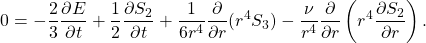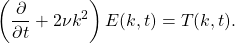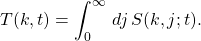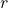## The energy balance equations: or what’s in a name?

Over the last few years I have noticed that the Karman-Howarth equation is sometimes referred to nowadays as the scale-by-scale energy budget equation’. Having thought about it carefully, I have concluded that I understand that description; but I think the mere fact that one has to think carefully is a disadvantage. To Anglophone speakers of English, the term budget’ suggests some sort of forward planning. Actually I think that in physics the more correct term would be local energy balance equation. Let us consider the form of the KHE equation when it is written in terms of the second-order and third-order structure functions, thus:Note that all notation and background for this post will be found in my (2014) book on HIT. Also, I have moved the term involving the total energy (per unit mass) to the right of the equal sign, for a reason which will become obvious.

More recently I have seen exactly the same phrase used to describe the Lin equation, which is just the Fourier transform of the KHE to wavenumber space. This strikes me as even more surprising, but again I don’t want to say that it is actually wrong. Indeed in one sense I rather welcome it, because it makes it clear that the concept of scale belongs equally to wavenumber space. It can be all too easy to fall into a usage in which real space is regarded as scale space’ and is distinguished in that way from wavenumber space. But the real problem here is that it is only valid for the simplest form of the Lin equation, and this in itself can be misleading.

Let us now consider the Lin equation in terms of the energy spectrum and the transfer spectrum. We may write this in its well-known form:Here, as with the KHE, we assume that there are no forces acting.

However, unlike the KHE, this is not the whole story. We may also express the transfer spectrum in terms of its spectral density, thus:When we substitute this in, we obtain the second form of the Lin equation, and this is actually more comparable with the KHE as given above, because the transfer spectrum density contains the Fourier transform of the third-order structure function, which of course occurs explicitly in the KHE.

Now compare the two equations. The KHE holds for any value of the independent variable. If we take some particular value of the independent variable, then each term can be evaluated as a number corresponding to that value of, and the above equation becomes a set of four numbers adding up to zero. If we consider another value of, then we have a different four numbers but they must still add up to zero. In short, KHE is local in the independent variable.

The Lin equation, if we write it in its full form, tells us that all the Fourier modes are coupled to each other. It is, in the language of physics, an example of the many body problem. It is in fact highly non-local as in principle it couples every mode to every other mode.

A corollary of this is that the KHE does not predict a cascade. But the Lin equation does. This can be deduced from the nonlinear term which couples all modes together plus the presence of the viscous term which is symmetry-breaking. If the viscous term were set equal to zero, then the coupled but inviscid equation would yield equipartition states.

The well-known question at the head of this post is rhetorical and expects the answer A rose by any other name would smell as sweet’. But I’m afraid that Juliet’s laissez-faire attitude to terminology would not be widely applicable. One thinks of the surgeon who fails to distinguish between the liver and the spleen. Or the pilot who thinks west is just as good a name for east. In the turbulence community, I suppose that locality’ for localness’, or inverse’ for reverse’ arise because they seem natural coinages to non-Anglophones. In the wider world, the classic case since the 1960s is Karl Popper’s idea that a scientific theory should be falsifiable. But in everyday English speech, to falsify means to make false. For instance, to falsify an entry in one’s accounts, means, to put it in the demotic, to cook the books!

I shall return to this point in future posts and in particular to the localness of the KHE.